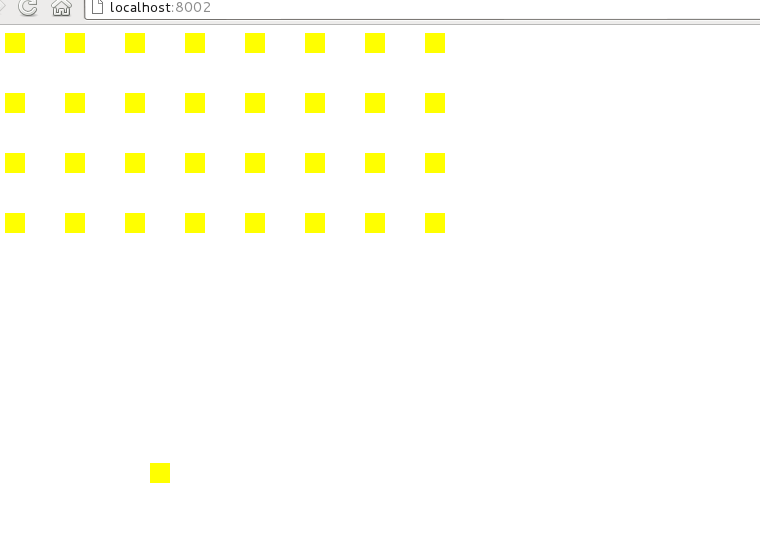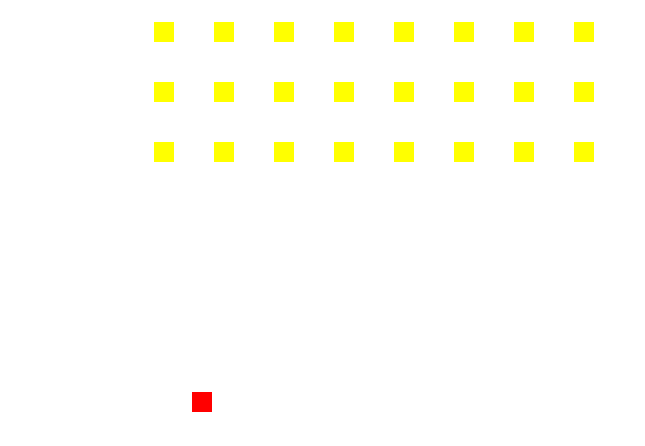# Learn functional programming with me - keyboard input for our red square

###### Published on 2013-6-13

Now we have some enemies, let's add our player and see how we might achieve the handling of keyboard events to move him.

Well, first off let's create the player

`` :player (initPlayer 0 430 20 20)``

Where

``````(defn initPlayer [x y w h]
{
:x x
:y y
:w w
:h h
}
)``````

There are some similarities here between the player and the enemy, and perhaps I'll address that when I'm a position to tell how.

Now, I'll want to render that player, and I'm actually going to take the bold step of pulling out a render function so my main loop now looks like this:

``````(defn tick [ctx state]
(clearScreen ctx)
(renderScene ctx state)
(js/setTimeout (fn []
(tick ctx (doLogic state))
) 33  )
)``````

Just keeping things tidy!

``````(defn renderScene [ctx state]
(enemiesRender ctx state)
)

(defn enemiesRender [ctx state]
(let [enemies (:enemies state)]
(doseq [enemy enemies]
(let [{:keys [x y w h]} enemy]
(drawSquare ctx x y w h)
)
)
)
)``````

Right, so now I have a player, I may as well render it

``````(defn renderScene [ctx state]
(enemiesRender ctx state)
(playerRender ctx state)
)

(defn playerRender [ctx state]
(let [player (:player state)]
(let [{:keys [x y w h]} player]
(drawSquare ctx x y w h)
)
)
)``````

And I'll need to copy it across to the new state each iteration

``````(defn doLogic [state]
{
:direction (directionLogic state)
:enemies (enemiesLogic state)
:player (:player state)
}
)``````

Nothing new here, this'll just give me an additional yellow square on my canvas...Handling those input events

Now I want to move it though, and this is where things are going to get fun, from what I can imagine off the top of my head (I'm currently sat on a plane at 35,000ft with no internet so I can't look anything up), my options are:

• Attempt to mutate player state directly as input events happen
• Keep some mutable state around to indicate whether certain keys are currently down, and apply these modifiers as part of logic

I think I'm going to opt for the second one and see how that plays out. It kinda makes sense to me in ignorant-land that isolating areas of mutable state from my (currently quite) clean program will be helpful.

This is pretty much what the structure will look like:

``````tick (state) =>
inputs = getCurrentInputs()
tick applyLogic(state, inputs)``````

I don't have to care that behind that getCurrentInputs is some horrible interop with the browser and piles of mutability, and if I work out how to do it better later it should limit the area of change needing to be applied.

I can hook the events using the standard js interop, which looks like this

``````(defn hookInputEvents []
(fn [e]
(setKeyState e.KeyCode true)
)
)
(fn [e]

)
)
)``````

Okay, so what now? I need to get this information to the game somehow, and actually - the information I want is "Is the key currently down", which is state I need to build up off those events.

Up and Atom

So I had to a bit of trawling to get this right, there is a notion of an "atom", in Clojure which is effectively a mutable object with some synchronisation over the top of it.

I've tried to avoid having any of this trickery yet, but this is somewhere I think going to need it.

So, an atom - I'm putting this into my 'global' scope (actually, it's namespaced into (ns game))

Define some state called keyStates, assign to it an atom (initial value is an empty map)

``(def keyStates (atom {}))``

Now, if I want to access the value of keyStates, I can de-rererence it thusly

De-reference an atom

``@keystates``

I also wanted to know how to "modify a single value of a map", for reasons that are about to become clear, the way to do this is

Create a new map, with a single property changed

``(assoc myMap :name newValue)``

This function returns a new map which is the same as the old one, but with that value changed (Hey, this is quite useful!)

The final piece of my jigsaw is the ability to change the value of this mutable atom, to do this I can use 'swap'

``(swap! myAtom (fn [oldValue] newValue))``

So how about that setKeyState function?

Set the new keyStates to be the same as the old keyStates, but with a new value for the current keycode

``````(defn setKeyState [code, value]
(swap! keyStates assoc code value)
)``````

Swap will call assoc, passing in the old state and the other args specified (hey, this is quite functional!)

Now I have a global state for my current input, which means I can ask questions of it and apply those answers to my state.

Applying input to my square

Well, I'll make a function whose job it is to take in the old state and return the new state and that seems to be working well for me so far.

``````(defn playerLogic [state]

)

(defn doLogic [state]
{
:direction (directionLogic state)
:enemies (enemiesLogic state)
:player (playerLogic state)
}
)``````

And in this, I'll work out from the input what to do to my state

``````(defn playerLogic [state]
(let [player (:player state)
left (@keyStates 37)
right (@keyStates 39)
]
(cond (= left true) (assoc player :x (dec (:x player)))
(= right true) (assoc player :x (inc (:x player)))
:else player
)
)
)``````

There is a whole lot to take where, but it boils down to

• Extract player from the state
• Extract 'left' from the de-referenced keyStates (37)
• Extract 'right' from the de-referenced keyStates (39)
• Decide what new state to return based on this value

Let's look at that last bit closer:

``````(cond (= left true) (assoc player :x (dec (:x player)))
(= right true) (assoc player :x (inc (:x player)))
:else player``````

This is kinda equivalent to

``````if(left) { }
else if(right) {}
else {}``````

The expressions on the RHS will be evaluated and returned if the LHS is true.

We've already looked at assoc, we recognise dec/inc and basically all I'm doing is saying, 'return a new player with x changed, here is the new value of x, it's a modification of the current x'

I bet there is a tidier way of doing this actually, maybe a built-in but I came up with

Please apply the specified function to the specified key in this map and return the result

``````(defn applyMod [m k func]
(assoc m k (func (m k)))
)``````

And in usage:

``````(cond (= left true) (applyMod player :x dec)
(= right true) (applyMod player :x inc)
:else player``````

Paint our player Red

I said red square, and so far it's yellow, let's fix this problem.

``````(defn drawSquare [[ctx width height] x y w h c]
(set! (. ctx -fillStyle) c)
(.fillRect ctx x y w h)
)

(defn enemiesRender [ctx state]
(let [enemies (:enemies state)]
(doseq [enemy enemies]
(let [{:keys [x y w h]} enemy]
(drawSquare ctx x y w h "#FF0")
)
)
)
)

(defn playerRender [ctx state]
(let [player (:player state)]
(let [{:keys [x y w h]} player]
(drawSquare ctx x y w h "#F00")
)
)
)``````

The drawing square function and repetition across my two types of entity still bugs me, but I figure I'll yet manage to tidy this up.

The result